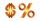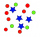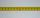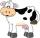# If we

If we increase the unknown number by 4%, we get 780. Determine the unknown number.

x =  750

### Step-by-step explanation:Did you find an error or inaccuracy? Feel free to write us. Thank you!Tips to related online calculators
Do you have a linear equation or system of equations and looking for its solution? Or do you have a quadratic equation?

## Related math problems and questions:

• Reducing numberReducing the an unknown number by 28.5% we get number 243.1. Determine unknown number.
• Percentage increaseIncrease number 400 by 3.5%
• Unknown number 716% of the unknown number is by 21 less than unknown number itself. Determine the natural unknown number.
• Unknown number 5I think of an unknown number. If we enlarge it five times, then subtract 3, and the result decreases by 75%, we get one greater than the number. What number am I thinking of?
• Cinema ticketsCinema sold 180 tickets this Thursday, which is 20%. Monday 14%, Tuesday 6%, Wednesday 9%, Friday 24%, Saturday 12%, and Sunday 15%. How many tickets were sold per week?
• Unknown number x5% from an unknown number equals the number 26. What is the unknown number?
• Jane classWhen asked how many students are in class, Jane said, if we increase the number of students in our class by a hundred % and then add half the number of students, we get 100. How many students are in Jane's class?
• Price increase 2xIf two consecutive times we increase the price of the product by 20%, how many % is higher final price than the original?
• Unknown number15% of the unknown number is 18 less than 21% of the same number. What is the unknown number?The product of the two numbers is 900. If we increase the lowest number by two, then its product will increase by 150. Determine both numbers.
• Percentages above 100%What is 122% of 185? What is the meaning of percentages above 100%?
• Test pointsIf you earned 80% of the possible 40 points, how many points did you miss to get 100%?
• The ratio 4The ratio of two number is 5:4 if 40% of the first number is 12, what will be 50% of the second number?
• Percent chainingCalculate 20% from 70% from 80 km
• Unknown number 24f we add 20, we get 50% of its triple. What is this unknown number?
• CowsAgricultural cooperative has increased the number of housed cows by 14% to 285 units. By how many cows increased agricultural cooperative the number of cows?
• Percents from percentHow much is 13% of 20% of 500 greater than 8% of 14% of 200?